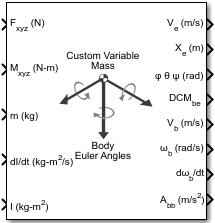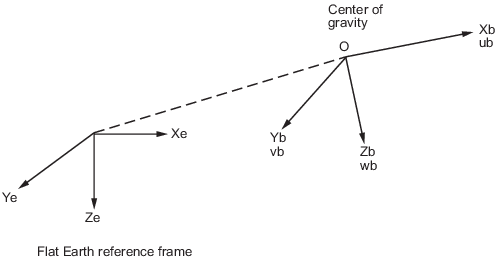# Custom Variable Mass 6DOF (Euler Angles)

Implement Euler angle representation of six-degrees-of-freedom equations of motion of custom variable mass

• Library:
• Aerospace Blockset / Equations of Motion / 6DOF

•## Description

The Custom Variable Mass 6DOF (Euler Angles) block implements the Euler angle representation of six-degrees-of-freedom equations of motion of custom variable mass. It considers the rotation of a body-fixed coordinate frame (Xb, Yb, Zb) about a flat Earth reference frame (Xe, Ye, Ze). For more information on Euler angles, see Algorithms.

## Limitations

The block assumes that the applied forces act at the center of gravity of the body.

## Ports

### Input

expand all

Applied forces, specified as a three-element vector.

Data Types: `double`

Applied moments, specified as a three-element vector.

Data Types: `double`

One or more rates of change of mass (positive if accreted, negative if ablated), specified as a three-element vector.

#### Dependencies

To enable this port, select Include mass flow relative velocity.

Data Types: `double`

Mass, specified as a scalar.

Data Types: `double`

Rate of change of inertia tensor matrix, specified as a 3-by-3 matrix.

Data Types: `double`

Inertia tensor matrix, specified as a 3-by-3 matrix.

Data Types: `double`

One or more relative velocities at which the mass is accreted to or ablated from the body in body-fixed axes, specified as a three-element vector.

#### Dependencies

To enable this port, select Include mass flow relative velocity.

Data Types: `double`

### Output

expand all

Velocity in the flat Earth reference frame, returned as a three-element vector.

Data Types: `double`

Position in the flat Earth reference frame, returned as a three-element vector.

Data Types: `double`

Euler rotation angles [roll, pitch, yaw], returned as a three-element vector, in radians.

Data Types: `double`

Coordinate transformation from flat Earth axes to body-fixed axes, returned as a 3-by-3 matrix.

Data Types: `double`

Velocity in body-fixed frame, returned as a three-element vector.

Data Types: `double`

Angular rates in body-fixed axes, returned as a three-element vector, in radians per second.

Data Types: `double`

Angular accelerations in body-fixed axes, returned as a three-element vector, in radians per second squared.

Data Types: `double`

Accelerations in body-fixed axes with respect to body frame, returned as a three-element vector.

Data Types: `double`

Accelerations in body-fixed axes with respect to inertial frame (flat Earth), returned as a three-element vector. You typically connect this signal to the accelerometer.

#### Dependencies

This port appears only when the Include inertial acceleration check box is selected.

Data Types: `double`

## Parameters

expand all

### Main

Input and output units, specified as `Metric (MKS)`, `English (Velocity in ft/s)`, or `English (Velocity in kts)`.

UnitsForcesMomentAccelerationVelocityPositionMassInertia
`Metric (MKS)` NewtonNewton-meterMeters per second squaredMeters per secondMetersKilogramKilogram meter squared
`English (Velocity in ft/s)` PoundFoot-poundFeet per second squaredFeet per secondFeetSlugSlug foot squared
`English (Velocity in kts)` PoundFoot-poundFeet per second squaredKnotsFeetSlugSlug foot squared

#### Programmatic Use

 Block Parameter: `units` Type: character vector Values: `Metric (MKS)` | `English (Velocity in ft/s)` | `English (Velocity in kts)` Default: `Metric (MKS)`

Mass type, specified according to the following table.

Mass TypeDescriptionDefault for
`Fixed`

Mass is constant throughout the simulation.

`Simple Variable`

Mass and inertia vary linearly as a function of mass rate.

`Custom Variable`

Mass and inertia variations are customizable.

The `Custom Variable` selection conforms to the previously described equations of motion.

#### Programmatic Use

 Block Parameter: `mtype` Type: character vector Values: `Fixed` | `Simple Variable` | ```Custom Variable``` Default: `'Custom Variable'`

Equations of motion representation, specified according to the following table.

RepresentationDescription

`Euler Angles`

Use Euler angles within equations of motion.

`Quaternion`

Use quaternions within equations of motion.

The `Quaternion` selection conforms to the equations of motion in Algorithms.

#### Programmatic Use

 Block Parameter: `rep` Type: character vector Values: `Euler Angles` | `Quaternion` Default: `'Euler Angles'`

Initial location of the body in the flat Earth reference frame, specified as a three-element vector.

#### Programmatic Use

 Block Parameter: `xme_0` Type: character vector Values: `'[0 0 0]'` | three-element vector Default: `'[0 0 0]'`

Initial velocity in body axes, specified as a three-element vector, in the body-fixed coordinate frame.

#### Programmatic Use

 Block Parameter: `Vm_0` Type: character vector Values: `'[0 0 0]'` | three-element vector Default: `'[0 0 0]'`

Initial Euler orientation angles [roll, pitch, yaw], specified as a three-element vector, in radians. Euler rotation angles are those between the body and north-east-down (NED) coordinate systems.

#### Programmatic Use

 Block Parameter: `eul_0` Type: character vector Values: `'[0 0 0]'` | three-element vector Default: `'[0 0 0]'`

Initial body-fixed angular rates with respect to the NED frame, specified as a three-element vector, in radians per second.

#### Programmatic Use

 Block Parameter: `pm_0` Type: character vector Values: `'[0 0 0]'` | three-element vector Default: `'[0 0 0]'`

Select this check box to add a mass flow relative velocity port. This is the relative velocity at which the mass is accreted or ablated.

#### Programmatic Use

 Block Parameter: `vre_flag` Type: character vector Values: `off` | `on` Default: `off`

Select this check box to add an inertial acceleration port.

#### Dependencies

To enable the Abe port, select this parameter.

#### Programmatic Use

 Block Parameter: `abi_flag` Type: character vector Values: `'off'` | `'on'` Default: `off`

### State Attributes

Assign a unique name to each state. You can use state names instead of block paths during linearization.

• To assign a name to a single state, enter a unique name between quotes, for example, `'velocity'`.

• To assign names to multiple states, enter a comma-separated list surrounded by braces, for example, `{'a', 'b', 'c'}`. Each name must be unique.

• If a parameter is empty (`' '`), no name is assigned.

• The state names apply only to the selected block with the name parameter.

• The number of states must divide evenly among the number of state names.

• You can specify fewer names than states, but you cannot specify more names than states.

For example, you can specify two names in a system with four states. The first name applies to the first two states and the second name to the last two states.

• To assign state names with a variable in the MATLAB® workspace, enter the variable without quotes. A variable can be a character vector, cell array, or structure.

Position state names, specified as a comma-separated list surrounded by braces.

#### Programmatic Use

 Block Parameter: `xme_statename` Type: character vector Values: `''` | comma-separated list surrounded by braces Default: `''`

Velocity state names, specified as comma-separated list surrounded by braces.

#### Programmatic Use

 Block Parameter: `Vm_statename` Type: character vector Values: `''` | comma-separated list surrounded by braces Default: `''`

Euler rotation angle state names, specified as a comma-separated list surrounded by braces.

#### Programmatic Use

 Block Parameter: `eul_statename` Type: character vector Values: `''` | comma-separated list surrounded by braces Default: `''`

Body rotation rate state names, specified comma-separated list surrounded by braces.

#### Programmatic Use

 Block Parameter: `pm_statename` Type: character vector Values: `''` | comma-separated list surrounded by braces Default: `''`

## Algorithms

The origin of the body-fixed coordinate frame is the center of gravity of the body. The body is assumed to be rigid, which eliminates the need to consider the forces acting between individual elements of mass. The flat Earth reference frame is considered inertial, an excellent approximation that allows the forces due to the Earth's motion relative to the “fixed stars” to be neglected.The translational motion of the body-fixed coordinate frame is given below, where the applied forces [Fx Fy Fz]T are in the body-fixed frame. Vreb is the relative velocity in the body axes at which the mass flow ($\stackrel{˙}{m}$) is ejected or added to the body-fixed axes.

`$\begin{array}{l}{\overline{F}}_{b}=\left[\begin{array}{c}{F}_{x}\\ {F}_{y}\\ {F}_{z}\end{array}\right]=m\left({\stackrel{˙}{\overline{V}}}_{b}+\overline{\omega }×{\overline{V}}_{b}\right)+\stackrel{˙}{m}\overline{V}r{e}_{b}\\ {A}_{be}=\frac{{\overline{F}}_{b}-\stackrel{˙}{m}{\overline{V}}_{r{e}_{b}}}{m}\\ {A}_{bb}=\left[\begin{array}{c}{\stackrel{˙}{u}}_{b}\\ {\stackrel{˙}{v}}_{b}\\ {\stackrel{˙}{w}}_{b}\end{array}\right]=\frac{{\overline{F}}_{b}-\stackrel{˙}{m}{\overline{V}}_{r{e}_{b}}}{m}-\overline{\omega }×{\overline{V}}_{b}\\ {\overline{V}}_{b}=\left[\begin{array}{c}{u}_{b}\\ {v}_{b}\\ {w}_{b}\end{array}\right],\overline{\omega }=\left[\begin{array}{c}p\\ q\\ r\end{array}\right]\end{array}$`

The rotational dynamics of the body-fixed frame are given below, where the applied moments are [L M N]T, and the inertia tensor I is with respect to the origin O.

`$\begin{array}{l}{\overline{M}}_{B}=\left[\begin{array}{c}L\\ M\\ N\end{array}\right]=I\stackrel{˙}{\overline{\omega }}+\overline{\omega }×\left(I\overline{\omega }\right)+\stackrel{˙}{I}\overline{\omega }\\ \\ I=\left[\begin{array}{ccc}{I}_{xx}& -{I}_{xy}& -{I}_{xz}\\ -{I}_{yx}& {I}_{yy}& -{I}_{yz}\\ -{I}_{zx}& -{I}_{zy}& {I}_{zz}\end{array}\right]\\ \\ \stackrel{˙}{I}=\left[\begin{array}{ccc}{\stackrel{˙}{I}}_{xx}& -{\stackrel{˙}{I}}_{xy}& -{\stackrel{˙}{I}}_{xz}\\ -{\stackrel{˙}{I}}_{yx}& {\stackrel{˙}{I}}_{yy}& -{\stackrel{˙}{I}}_{yz}\\ -{\stackrel{˙}{I}}_{zx}& -{\stackrel{˙}{I}}_{zy}& {\stackrel{˙}{I}}_{zz}\end{array}\right]\end{array}$`

The relationship between the body-fixed angular velocity vector, [p q r]T, and the rate of change of the Euler angles, ${\left[\stackrel{˙}{\varphi }\stackrel{˙}{\theta }\stackrel{˙}{\psi }\right]}^{\text{T}}$, can be determined by resolving the Euler rates into the body-fixed coordinate frame.

 `$\left[\begin{array}{c}p\\ q\\ r\end{array}\right]=\left[\begin{array}{c}\stackrel{˙}{\varphi }\\ 0\\ 0\end{array}\right]+\left[\begin{array}{ccc}1& 0& 0\\ 0& \mathrm{cos}\varphi & \mathrm{sin}\varphi \\ 0& -\mathrm{sin}\varphi & \mathrm{cos}\varphi \end{array}\right]\left[\begin{array}{c}0\\ \stackrel{˙}{\theta }\\ 0\end{array}\right]+\left[\begin{array}{ccc}1& 0& 0\\ 0& \mathrm{cos}\varphi & \mathrm{sin}\varphi \\ 0& -\mathrm{sin}\varphi & \mathrm{cos}\varphi \end{array}\right]\left[\begin{array}{ccc}\mathrm{cos}\theta & 0& -\mathrm{sin}\theta \\ 0& 1& 0\\ \mathrm{sin}\theta & 0& \mathrm{cos}\theta \end{array}\right]\left[\begin{array}{c}0\\ 0\\ \stackrel{˙}{\psi }\end{array}\right]={J}^{-1}\left[\begin{array}{c}\stackrel{˙}{\varphi }\\ \stackrel{˙}{\theta }\\ \stackrel{˙}{\psi }\end{array}\right]$`

Inverting J then gives the required relationship to determine the Euler rate vector.

`$\left[\begin{array}{c}\stackrel{˙}{\varphi }\\ \stackrel{˙}{\theta }\\ \stackrel{˙}{\psi }\end{array}\right]=J\left[\begin{array}{c}p\\ q\\ r\end{array}\right]=\left[\begin{array}{ccc}1& \left(\mathrm{sin}\varphi \mathrm{tan}\theta \right)& \left(\mathrm{cos}\varphi \mathrm{tan}\theta \right)\\ 0& \mathrm{cos}\varphi & -\mathrm{sin}\varphi \\ 0& \frac{\mathrm{sin}\varphi }{\mathrm{cos}\theta }& \frac{\mathrm{cos}\varphi }{\mathrm{cos}\theta }\end{array}\right]\left[\begin{array}{c}p\\ q\\ r\end{array}\right]$`

 Stevens, Brian, and Frank Lewis. Aircraft Control and Simulation, 2nd ed. Hoboken, NJ: John Wiley & Sons, 2003.

 Zipfel, Peter H. Modeling and Simulation of Aerospace Vehicle Dynamics. 2nd ed. Reston, VA: AIAA Education Series, 2007.

## Support평가판 신청